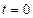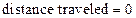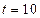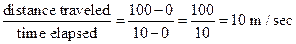# To find the average speed of each winner.### Precalculus: Mathematics for Calcu...

6th Edition
Stewart + 5 others
Publisher: Cengage Learning
ISBN: 9780840068071### Precalculus: Mathematics for Calcu...

6th Edition
Stewart + 5 others
Publisher: Cengage Learning
ISBN: 9780840068071

#### Solutions

Chapter 2.4, Problem 31E

a.

To determine

## To find the average speed of each winner.

Expert Solution

The average speed of each of the winner is 10 m/sec .

### Explanation of Solution

Given information:

The graph provided in the question shows the distance as a function of time for each of the three winners.

Calculation:

Since, each of the winner reached finished the race at the same time that is at t=10 sec.

So, the average speed of each of the winner must be same.

Therefore,

At t=0 sec, distance traveled=0 m.

At t=10 sec, distance traveled=100 m.

Average speed of each of the winner is obtained as:

distance traveledtime elapsed=1000100=10010=10 m/sec

Hence,

The average speed of each of the winner is 10 m/sec .

b.

To determine

### To explain the difference between the ways in which the three runners ran the race.

Expert Solution

The differencebetween the ways in which the three runners ran the race is that the runner A ran the first half of the race with a higher speed that is 16 m/sec and in the next half, he slow down to 5 m/sec , runner C ran the first half of the race with a relatively lesser speed that is 5 m/sec and in the next half, he boast up to 16 m/sec , and runner B ran the whole race at an average speed of 10 m/sec .

### Explanation of Solution

Given information:

The graph provided in the question shows the distance as a function of time for each of the three winners.

The graph showing the distance travelled by the runner at different time interval.

Since, each of the winner reached finished the race at the same time that is atSo, the average speed of each the winner must be same

Therefore

At, t-0 sec,m.

Atsec, distance traveled-100 m.

Average speed of each of the winner is obtained asHence,

The average speed of each of the winner is 10m/sec.From the above graph, it can be observed that, distance travelled by A at t=5 sec is 80 m, distance travelled by B at t=5 sec is 50 m and distance travelled by C at t=5 sec is 20 m.

Time elapsed =5 sec

Average speed of runner A from 0 to 5 second is obtained as:

distance travelledtime elapsed=805=16 m/sec

Average speed of runner B from 0 to 5 second is obtained as:

distance travelledtime elapsed=505=10 m/sec

Average speed of runner C from 0 to 5 second is obtained as:

distance travelledtime elapsed=205=4 m/sec

Similarly,

Average speed of runner A from 5 to 10 second is obtained as:

distance travelledtime elapsed=100805=205=5 m/sec

Average speed of runner B from 5 to 10 second is obtained as:

distance travelledtime elapsed=100505=505=10 m/sec

Average speed of runner C from 5 to 10 second is obtained as:

distance travelledtime elapsed=100205=805=16 m/sec

Hence,

It can be concluded easily that the differencebetween the ways in which the three runners ran the race is that the runner A ran the first half of the race with a higher speed that is 16 m/sec and in the next half, he slow down to 5 m/sec , runner C ran the first half of the race with a relatively lesser speed that is 5 m/sec and in the next half, he boast up to 16 m/sec , and runner B ran the whole race at an average speed of 10 m/sec .

### Have a homework question?

Subscribe to bartleby learn! Ask subject matter experts 30 homework questions each month. Plus, you’ll have access to millions of step-by-step textbook answers!